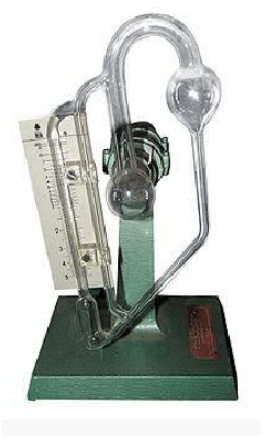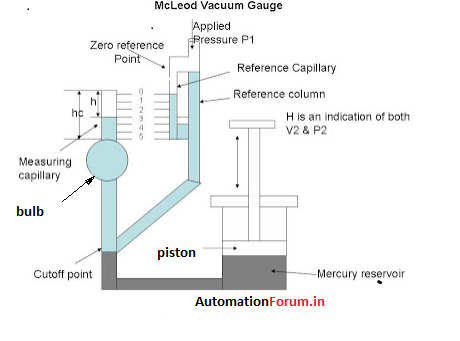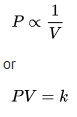# How to measure pressure using a McLeod gauge?

McLeod gauge is a commonly used device for low-pressure measurements. It is essentially a manometer which uses mercury as the manometric fluid. It can be used for measuring pressures up to 0.1 torr. It was invented by Herbert McLeod in 1874.## The process of measurementThe basic form of a McLeod gauge is shown above. It operates by taking a sample of gas in a vacuum chamber and then compressing it with mercury.

Initially, the pressure to be measured is applied to the reference column. Then piston is withdrawn from the mercury reservoir, lowering the level of mercury below the cutoff point, thereby admitting the gas of unknown pressure P1. Let the volume of gas in the measuring capillary and the bulb be V1.

When the piston is pushed in, the mercury level goes up sealing the unknown volume of gas sample in the measuring capillary and the bulb. Then the motion of the plunger is continued until the level of mercury reaches the zero level in the reference capillary as shown in the figure. The volume of the gas is measured from the scale. The difference in height “h” of measuring capillary and reference capillary is the measure of volume V1 and the pressure P2.

## How to calculate the unknown pressure?

The unknown pressure is determined by using Boyle’s Law.

Boyle’s Law states that the absolute pressure exerted by a given mass of an ideal gas is inversely proportional to the volume it occupies if the temperature and amount of gas remain unchanged within a closed system.

Mathematically, Boyle’s law can be stated as Pressure is inversely proportional to the volume.where P is the pressure of the gas, V is the volume of the gas, and k is a constant.

V1 is the initial volume of the gas trapped in the bulb and the measuring capillary and V2 is the final volume of the gas. P1 is the applied pressure to be measured. And let the area of the cross-section be “a”. Therefore,

V1=V+a*hc

V2=a*h

P2 is the final pressure

P2=P1+h

So according to Boyle’s law,

P1V1 =(P1+h)(a*h)

P1V1 =P1ah+a(h^2)

P1 = a(h^2)/(V1-ah) ah is very small

P1 =a(h^2)/V1